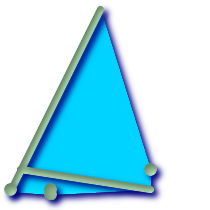## Name

ST_ConvexHull — The convex hull of a geometry represents the minimum convex geometry that encloses all geometries within the set.

## Synopsis

`geometry ST_ConvexHull(`geometry geomA`)`;

## Description

The convex hull of a geometry represents the minimum convex geometry that encloses all geometries within the set.

One can think of the convex hull as the geometry you get by wrapping an elastic band around a set of geometries. This is different from a concave hull which is analogous to shrink-wrapping your geometries.

It is usually used with MULTI and Geometry Collections. Although it is not an aggregate - you can use it in conjunction with ST_Collect to get the convex hull of a set of points. ST_ConvexHull(ST_Collect(somepointfield)).

It is often used to determine an affected area based on a set of point observations.

Performed by the GEOS moduleThis method implements the OpenGIS Simple Features Implementation Specification for SQL 1.1. s2.1.1.3This method implements the SQL/MM specification. SQL-MM 3: 5.1.16This function supports 3d and will not drop the z-index.

## Examples

```--Get estimate of infected area based on point observations
SELECT d.disease_type,
ST_ConvexHull(ST_Collect(d.the_geom)) As the_geom
FROM disease_obs As d
GROUP BY d.disease_type;
```Convex Hull of a MultiLinestring and a MultiPoint seen together with the MultiLinestring and MultiPoint

```SELECT ST_AsText(ST_ConvexHull(
ST_Collect(
ST_GeomFromText('MULTILINESTRING((100 190,10 8),(150 10, 20 30))'),
ST_GeomFromText('MULTIPOINT(50 5, 150 30, 50 10, 10 10)')
)) );
---st_astext--
POLYGON((50 5,10 8,10 10,100 190,150 30,150 10,50 5))
```